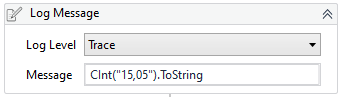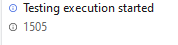# Convert to integer error

hello, I have a string expression as follows;

a(String)=15,05

I want to convert it to integral, but I want to include the decimal side, for example;

new a(int): 15,05

Hello @d.ulutas

I looked but couldn’t find what I was looking for.

Hey @d.ulutas

Integer can’t have comma with it.

Do you want it to be considered as decimal ?

Thanks
#nK

yes it does. I will do the subtraction at the end.

1 Like

Hey @d.ulutas

Try this then,

``````CDbl(a.Replace(",","."))
``````

Thanks
#nK

you can first replace the comma using Rplace command and then do the substration. Then using format value activity you can add the comma (By providing the format that you require)

Hello.

An integer cannot have a decimal point.
The ideal is to convert to decimal but first you must convert the comma to a point.

CDbl(a.Replace(“,”,“.”))

Hug

Thank you for your answers, but I couldn’t find exactly what I was looking for;

There are two counts;

a: 25.008,00
b: 25.008,01

I want to find the price difference between them. But if I do a-b it will get -0.01. So I have to do b-a. This order may change tomorrow, for example;

a: 25.008,01
b: 25.008,00

How can I do that ?

Hello.

Before doing the math, you have to see which number is higher among them.

Okey but How ?

Convert values to decimal.

Then use an if and validate
IF- (A > B)
Then: the account will be A-B
Else B-A

and these expressions are strings. I couldn’t convert them.

Use CInt. (I just put the ToString on there to spit the value back out, but you don’t need the ToString)a: 25.008.00
b: 25.08.01

they don’t turn

Because? It’s simple!

double.parse(yourvalue.replace(“.”,“”).replace(“,”,“.”))

He wants to convert to integer, not double.

I know, but an integer doesn’t work for numbers with commas. That’s why I suggested decimal.

no dont work. because 25(.) dont work

double.parse(değeriniz.replace(“.”,“”).replace(“,”,“.”)) ---->

25008,00

but i want

25.008.00